# Convert Scientific Notation to Text or a Number in Excel

This post will guide you how to convert scientific notation to text or a number in Excel. How do I convert excel scientific to text with a formula in Excel.

## Convert Scientific Notation to Text or a Number in Excel

Assuming that you have a list of data in range B1:B4 that contain scientific notation numbers and you want to convert those scientific number to text or an integer number. How to achieve it.

You can use the Format cell feature to achieve the result. Just do the following steps:

#1 select the range of cells that you want to convert.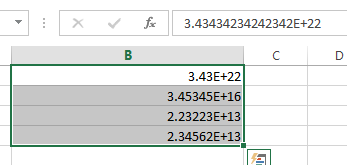#2 right click on it, and select Format Cells from the pop-up menu list. And the Format Cells dialog will open.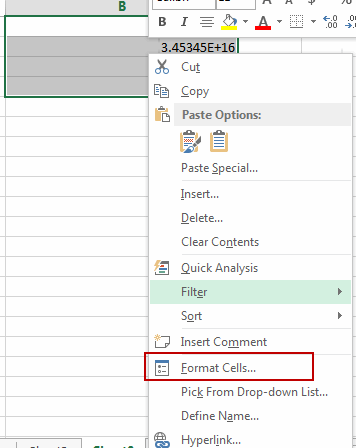#3 switch to Number tab, click Custom under Category list box. And type 0 in the type text box. Then click OK button.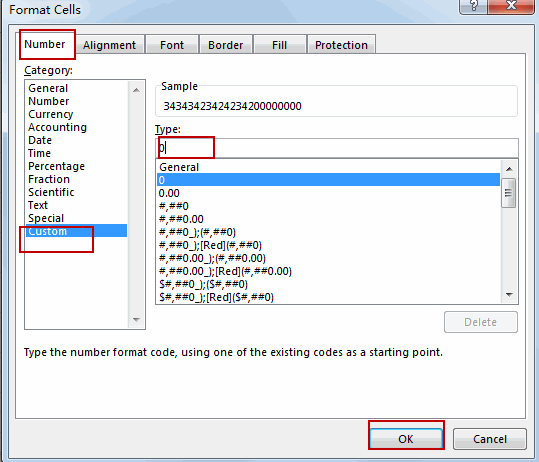#4 Let’s see the result: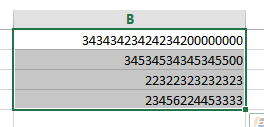You can also use an excel formula to convert scientific notation to text based on the TRIM function or the UPPER function.

Type the following formula into a blank cell and then press Enter key.

x
How to Select Every Other Row in Excel

=TRIM(B1)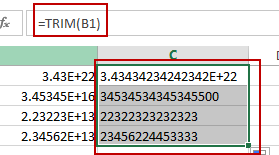Or

=UPPER(B1)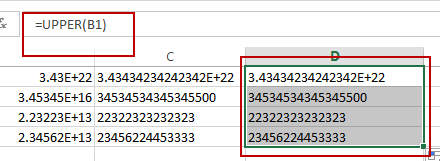There is a simple method to convert scientific notation to text, you just need to type a single quote before the scientific notation number. Then it will show the normal number.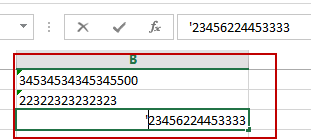### Related Functions

• Excel TRIM function
The Excel TRIM function removes all spaces from text string except for single spaces between words.  You can use the TRIM function to remove extra spaces between words in a string.The syntax of the TRIM function is as below:= TRIM (text)….
• Excel UPPER function
The Excel UPPER function converts all characters in text string to uppercase.The UPPER function is a build-in function in Microsoft Excel and it is categorized as a Text Function.The syntax of the UPPER function is as below:= UPPER (text)…
Related Posts

How To Convert Text to Upper Cases(Using VBA) in Excel

This post will show you how to switch from lower case to upper case in Excel. and I am going to show you two different ways of converting text to upper cases using formula or VBA macro in Excel 2013,Excel ...

How to Convert Uppercase to Lowercase Except the First Letter in Excel

In excel, words can be entered in uppercase, lowercase or mixed. If we want to convert all uppercase to lowercase (except the first letter in some situations), we can use formula with some letter convert related functions to convert them ...

How To Force Text String to Upper,Lower, or Proper Case in Excel

This post will guide you how to force test strings to uppercase or lowercase or Proper cases through an Excel formula or data validation in your Excel 2013/2016. How to convert text to upper, lower, or proper case in Excel. ...

How to Check If a Cell is Uppercase, Lowercases or Capitalize in Excel

This post will guide you how to check if a cell is uppercase in Excel 2013/2016. How do I identify all letters in cells are lowercases or capitalize in Excel. How to change letters to uppercase, lowercases or capitalize first ...

Change Uppercase and Lowercase Text

This post will guide you how to change the case of text to uppercase or lowercase in Excel. How do I change all uppercase text to lower case with an Excel formula. For example, if you want to change all ...

Remove Spaces from a String

This post will guide you how to remove extra spaces between words or text string from the specified range of cells in excel. How to delete all spaces from excel cells. For example, when you paste data from an external ...

In this tutorial you will learn that how to remove leading or trailing spaces from a text string in a cell in Excel. This post will guide you how to delete leading or trailing spaces of all cells in a ...

How to Count Spaces before the Text String

This post will guide you how to count the number of spaces before the text string in a cell in Excel. How to count the leading spaces before the text string in one cell with formula in excel. How do ...

How to Check If the First Letter is capitalized

This post explains that how to check if the first letter in a cell is capitalized or not in excel. How to write an excel Macro to check if the first letter in a specified cell is capitalized or not. ...

How to extract nth word from text string

This post will guide you how to extract the nth word from a text string in excel. How do I get the nth word from text string in a cell using excel formula. How to write a User Defined Function ...

Sidebar